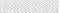# Python Generators: When and How

## Also Featuring: What and Why

When you write code, primarily, you want for it to work, and secondarily, you want for it to work efficiently. Efficient code minimizes those situations where you’re sitting there waiting for your script to run, wondering if you could have written more efficient code and spent the extra time sleeping, dreaming of rainbow unicorns. Efficient code is also just less likely to fail. We like that.

For example, sometimes, it’s better to use generator expressions than list comprehensions in Python—especially when you don’t need the list implicitly created by a list comprehension.

For example, if you were to run a list comprehension squaring numbers a sum function like this one:

`final_sum = sum([number ** 2 for number in range(100000000)])`

Python would run through the above code inside-out, sort of like this:Just the Beginning of the Massive List of Numbers you Never Needed to Make

Is there a more efficient way to do the same thing?

Yes! With a generator expression:

`final_sum = sum(number ** 2 for number in range(100000000))`

Notice the subtle difference from the list comprehension: We’ve ditched the square brackets. Now we’re just in the parenthetical brackets of the sum function, using an anonymous generator expression. Here’s the new order of operations this will follow:

Much better! We never had to save any list of integers into memory: We just lazily iterated through each integer from zero to a hundred million, adding each integer’s square to the previous sum we had saved. No list involved.

This simple example generator function is only ~7% faster at working through 100 million integers than a list comprehension doing the same task, but I’ll take that ~7% speed boost every time.

The list comprehension works for this task, but now that you know there’s a better, faster way to do the same thing, you will have a guilty conscience every time you go the slower, less efficient route, or at least I will.

When I attempt to sum the squares of a billion integers using the same functions, my jupyter kernel just crashes on the list comprehension. I don’t have enough memory to handle a list that huge. The generator doesn’t have that problem: It takes a good four minutes to run over a billion integers for me, but it runs. It doesn’t need nearly as much space in memory to do what it needs to do.

## But…What Even Is a Generator in Python?

Fair question. Now that we know we want to use it, let’s talk about what it is.

The following knowledge and examples draw heavily from Programiz:

Generators are great for another big reason: They can operate on an infinite or continuous stream of data. For example, the following function could, theoretically, keep returning even numbers forever by using an infinite while loop and a yield statement in tandem:

`### Define a generator function: ### def all_even_generator():    n = 0    while True: ### Aka always, forever;        yield n ### This is where it will yield and freeze;        n += 2  ### It starts up again here when we call next()### Instantiate a generator object: ###genny = all_even_generator()### Pump out some yields: ###next(genny)`

Recognize that this function doesn’t attempt to generate every even number in existence and return that as a list (which is impossible, there are infinite), it just gives us the next even number every time we call next() on the `genny` object.

## Conclusion

Use simple generator expressions instead of list comprehensions whenever you won’t need the actual list later.

Use formal generators when you need: A function that magically freezes itself after it yields something (which is like returning something), and can be un-frozen to continue yielding more things later.

Data Scientist // @cinemarob1

## More from Robert Boscacci

Data Scientist // @cinemarob1